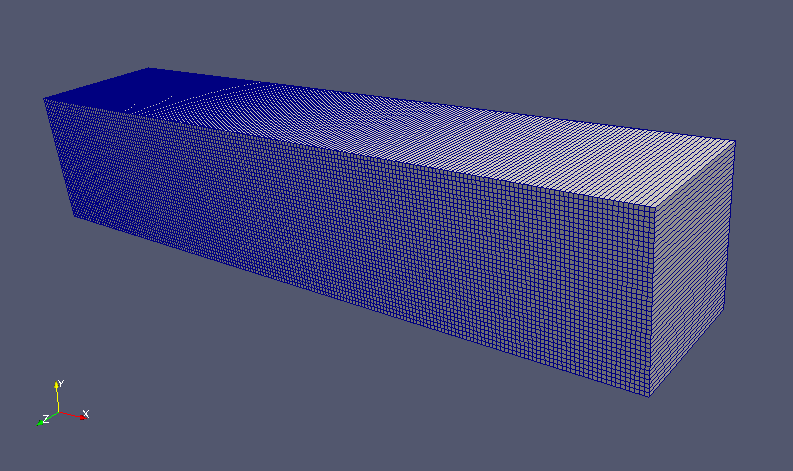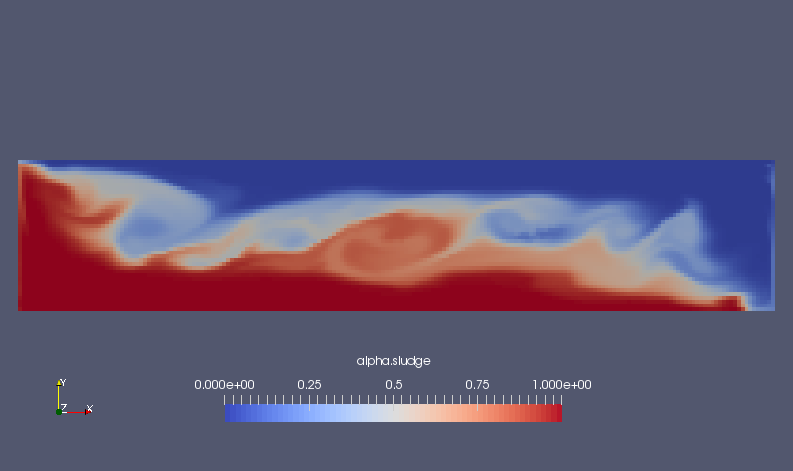﻿ Mixing of two types of liquids - XSim

# Mixing of two types of liquids

Update: July 10, 2017
OpenFOAM 4.x

## Case directory

\$FOAM_TUTORIALS/multiphase/twoLiquidMixingFoam/lockExchange

## Summary

We calculate the mixing of two liquids that have different densities for 10 seconds. There is no inflow or outflow of fluid into the analysis domain, and the boundary wall is assumed to be a slip wall, and the calculation is performed as a two-dimensional problem with one mesh in the Z direction.Model geometry

The gravity acceleration is defined as the negative direction on the Y-axis in the file constant/g.

```dimensions      [0 1 -2 0 0 0 0];
value           (0 -9.81 0);
```

The properties of each phase are set in the file "constant/transportProperties" as follows where nu is the kinematic viscosity (m2/s) and rho is the density (kg/m3).

```Dab           [0 2 -1 0 0 0 0]    1e-06;
alphatab      [0 0 0 0 0 0 0]     1;

phases (sludge water);

sludge
{
transportModel  Newtonian;
nu              [0 2 -1 0 0 0 0] 1e-06;
rho             [1 -3 0 0 0 0 0] 1000;
}

water
{
transportModel  Newtonian;
nu              [0 2 -1 0 0 0 0] 1e-06;
rho             [1 -3 0 0 0 0 0] 990;
}
```

The meshes are as follows, and the number of mesh is 8000.Meshes

The calculation result is as follows.

Volume ratio of sludge (alpha.sludge)Volume ratio of sludge at start time (alpha.sludge)Volume ratio of sludge at end time (alpha.sludge)

## Commands

cp -r \$FOAM_TUTORIALS/multiphase/twoLiquidMixingFoam/lockExchange lockExchange
cd lockExchange

rm -rf 0
cp -r 0.orig 0

blockMesh
setFields

twoLiquidMixingFoam

paraFoam

## Calculation time

53.43 seconds *Single, Inter(R) Core(TM) i7-2600 CPU @ 3.40GHz 3.40GHz